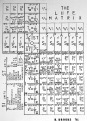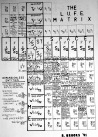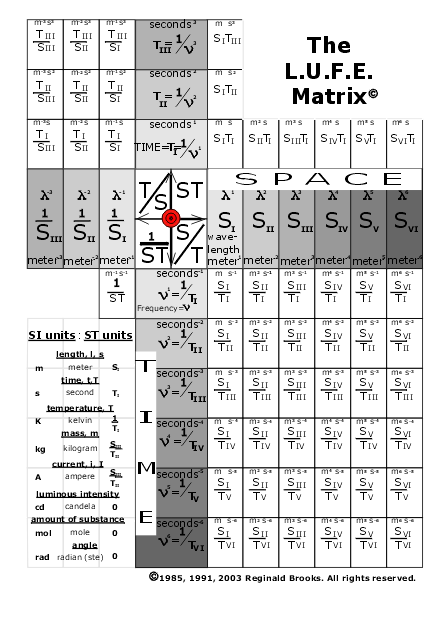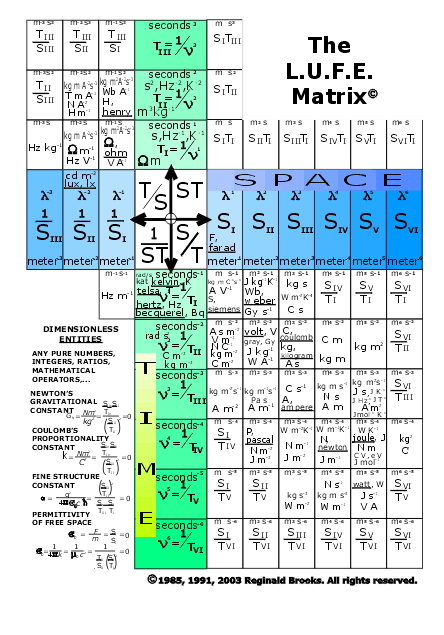art theory 101~ The LUFE Matrix: distilling SI quantities into ST dimensions

The LUFE Matrix:

The distillation of System International (SI) units into more fundamentally base units of Space-Time (ST) dimensions

Short Title: The Multi-dimensional LUFE Matrix

Introduction-Geometry (Layout)-Page 6a

Contents

Geometry

SI Units                                                                          page 6d

SI Quantities                                                                                page 6e

NOTE: The LUFE Matrix was developed and published by the author originally in 1985 and was subsequently presented in other papers culminating in the 1991-92 work from which the title and substance of this digitized version is based.These drawings are from that paper.

(click to enlarge images)

This digitized version of The LUFE Matrix supplements "Dark matter=Dark energy (the inversion of): The Conservation of SpaceTime by the Conservation of Force" by the author -see Page 5 of Art Theory 101.

Abstract (original extended 1995)

Apples to oranges. Dimensional analysis of physical entities (quantities) and the units used to express them has led to the formation of a clear and visually powerful ordering Space-Time (ST) Matrix, The L.U.F.E. (LUFE) Matrix.

The international system of units (SI)SI base, derived and supplementaryhas been systematically organized into the more fundamentally base units of space and time dimensions. This distillation of all physical entities and the units used to express them into common ST interactive dimensional units allows for a direct, visual display of the conceptual and mathematical operations which inform so much of scientific thought. A useful and dynamic tool for teaching, research and theory has been formed by reducing apples and oranges to ST units.

The conservation laws, in relation to the multidimensional LUFE Matrix, are examined in terms of the symmetry of spatial and temporal dimensional units. Conservation of spactime is proposed to be the fundamental symmetry of Nature.

The LUFE Matrix's reduction of SI units into ST dimensions coincides with the translation of the abstract mathematical symbolism into a readily visualized geometry of areasareas differentiated by common graphic means of outline, color, shading, etcwhich are manipulated in the LUFE Matrix by simple rules of addition and subtraction.

It is a new math (dynamic geometry) that is easy to learn, easy to see and easy to utilize as a tool to teach pure mathematics. Once combined with standard and theoretical concepts in physics, the LUFE Matrix offers a new window forward and mirror back into the cosmos.

A valuable supplement containing over 200 examples and proofs (some 97 pages, 216 figures on paper) of the LUFE Matrix reviewing classical and modern physics is available.(Digital version coming.)

Abstract extension (added July, 2003)

Any grand unification of the gravitational force (interaction) with the strong-electro-weak force (interaction) will require coming to terms with our most basic, fundamental understanding of the nature of mass, charge, spin and space-time itself, and how these identifying properties of matter are formed, interact and are conserved by the very space-time dimensions of which they are inseparably generated from.

Relativistic quantum theory will be clarified as we recast our eclectically derived notions of physical law into the base, fundamental units of space and time, or space-time dimensions.

The LUFE Matrix is the rosetta stone of physics. It visually translates our knowledge of the universe, and the mathematics used to describe it, into a simpler, more beautiful form.

The simplicity of that form belies its amazing dynamics. And while the body of the LUFE Matrix carries the fixed, reference components, it is in the wings that the dynamical lift is to be found.

Here, in flight, is where the LUFE Matrix can visually take one to both the past and the future. The LUFE Matrix Supplement: Examples and Proofs, will dynamically fly through the history of classical and modern physics, giving proof to its validity by example. It is a short course in its own right.

Dark-Dark-Light: Dark Matter=Dark Energy (the inversion of ): The Conservation of SpaceTime by the Conservation of Force (and Energy) dynamically flys one into the future. Here is a new theory which uses the LUFE Matrix to reinterpret the Standard Model Theory of the cosmos in terms of a more fundamental conservation law: the Conservation of Space-Time. The LUFE Matrix, as a rosetta stone...a visual theory of space-time dimension...is given wings that no sun can melt.

In closing, I have included five new constants from my own work in this realm...LUFE, The Layman's Unified Field Expose.

Page 6a- Geometry- Layout

Introduction

The evolution of our understanding of the physical world has been anything but a linear plot. Progress has occurred in leaps and bounds. The authenticity to which partial truths gain a stranglehold on the true progression of an idea is both bewildering and unfortunate. But fortunately, it is in our nature to inquire, to search, and to go deeper and deeper into a question to reach for the solution to that idea. The idea is nothing short of understanding the universe and our place in it.

From the concepts of fire, water, earth, and air as the fundamental interactive elements defining our world, we have traveled the winding road of scientific thought to the present in which the very space and time to which we have accumulated an enormous physical context into which our physical entities and their expressions exist, and have now come to realize the fundamental role they have in the actual formation of the physical world. Space and time do not exist separately and certainly not absolutely in any way from the matter and fields which have previously so dominated our search toward the idea. From Newtonian three-dimensional and absolute space and absolute time we have traveled, and not without enormous effort, to the Einsteinian four-dimensional, relative space-time and hence to our present and most far-reaching efforts.

Post-Einsteinian thought increasingly favors a multi-dimensional universe consisting of a number of spatial dimensions (nine or more) and a temporal dimension to give a nonabsolute 10+dimensional space-time fabric that includes all matter and all fields. Inherent in this approach is the integral roles that space and time play in the actual formation and subsequent expression of all physical phenomena. Such dimensional analysis may be enhanced and clarified once we have found the proper relationship(s) between these multiple dimensions of space and time. The LUFE Matrix is the tool.

The LUFE Matrix (L.U.F.E. stands for Layman's Unified Field Expose, a work published by the author in 1985, which describes in detail the development of the concepts which resulted in the formation of the LUFE Matrix) was developed in response to a need to compare apples with oranges. The International System of units (SI), which is used in the LUFE Matrix, itself is a step toward unifying the conceptual basis of physical thought by standardizing the units of physical expression. Dimensional analysis in the SI system allowed numerous and widely differing physical entities to be expressed into a small number (7) of SI base units (length, mass, time, electric current, temperature, molecular amounts and luminous intensity) and from these a much longer list of SI derived and supplementary units. The LUFE Matrix is a further distillation of this process. All SI quantities and units are expressed in fundamental space-time (ST) units (dimensions). This results in a great simplification of an enormous body of units (a simplification not unlike Mendeleev's Periodic Table of the Elements) into which a remarkable visual order is given to such an eclectic array of physical concepts, quantities and units. The LUFE Matrix is the rosetta stone of Nature. Use it to advance peace and harmony.

 ~~~~~~~~~~~~~~~~~~~~~~~~~~~~~~~~~~~~~~~~~~~~~~~~~~~~~~~~~~~~~~~~~~~~~~~~~~~~~~ sidebar 1: PREVIEW~~~~~~~~~~~~~~~~~~~~~~~~~~~~~~~~~~~~REVIEW can you say "Energy" ten different ways Slide Show Quick & Simple Visual Overview Recommended: Before & After ~~~~~~~~~~~~~~~~~~~~~~~~~~~~~~~~~~~~~~~~~~~~~~~~~~~~~~~~~~~~~~~~~~~~~~~~~~~~~~

The LUFE Matrix Layout

We start with the familiar x-, y-axis of the Cartesian coordinate system in which four quadrants are formed, designated as: ST, S/T, 1/(ST), and T/S, clockwise. More on this in a bit.

The horizontal x-axis designates the pure spatial dimensions:

Space (S) as SI, SII, SIII,and so on.

The vertical y-axis designates the pure temporal dimensions:

Time (T) as TI, TII, TIII,and so on.

From the origin, the spatial axis is positive to the right, negative to the left. The temporal axis is positive up, negative down.

Some entities have purely spatial dimensions, e.g., displacement, length, radius, area, volume, wavelength, etc. These are located on the horizontal "space" axis.

Some entities have purely temporal dimensions such as elapsed time or frequency, the two of which are reciprocally related in that frequency is cycles per second. (The inverse of time, T =1/T=n = frequency.) These are located on the vertical "time" axis.

However, the vast majority of physical entities are expressed in the interactive ST dimensions in which a combination of horizontal spatial dimension(s) and vertical temporal dimension(s) gives the physical expression. This appears on the LUFE Matrix in one of the four quadrants, usually in the S/T lower right quadrant as most entities have a net dimensional expression of so many S dimensions per so many T dimensions. For example, the velocity of light, c equals so much displacement per unit of time = meters per second = space/time = S/T= wavelength (l ) times frequency (n ) = l n .

When an item is per something spatial, or per something temporal, that is effectively dividing that item by the spatial or temporal dimension(s). Meters per second translate as m/s, or positive space meters and negative time seconds on the LUFE Matrix. This would place velocity, or m/s in the lower right quadrant as SI/TI (it is equivalent to placing an item on the x-, y- coordinate system at x=+1, y=-1). Another common way of expressing division of, or the denominator in a fraction, is with the negative exponential, i.e. m/s = m s-1 and m/s2 = m s-2, and so on.

That's it, that's just about as tough as it gets. We are going to refine this process and lay down some "rules of the matrix", but the idea is no more complicated than this. To avoid confusion of so much information, it is helpful to focus on the S/T quadrant realizing that the whole matrix is symmetric.

Notice that we have used the word dimension in three ways: (a) as spatial dimension, S; (b) as temporal dimension, T; and (c) as space-time dimension, ST. From the origin, the number of spatial dimensions increases sequentially as SI, SII, SIII,and so on, each S unit represents one unit of spatial dimension.

And yes, while we can readily assign any one unit of S (SI) as linear space, and two units of S (SII) as area, and any three units of S (SIII) as volume, we must accept Nature's design in which four (SIV) and five (SV) or more spatial dimensions are required.

The same for the temporal dimension, each increases sequentially out form the origin as 1/T I, 1/TII, 1/TIII,and so on. Here again, one unit of time may be thought of as per second, and two units as per second second, or per second 2, and so on.

Each pure space or pure time dimension (i.e., SI, SII, SIII, and 1/TI, 1/TII, 1/TIII,etc.) is to be thought of as extending linearly to infinity (like a beam of light) and at a direction perpendicular to its axial location. Thus SI extends vertically to infinity in both the positive and negative direction and 1/TI extends horizontally to infinity in both the negative and positive direction.

It is on the S/T quadrant of the matrix that the pure spatial and pure temporal dimensions overlapcrossoverforming an area of ST, here as S/T, that defines dynamic, interactional ST dimension. Here is where most of the physical entities express themselves. In the ongoing example, the linear SI dimension dynamically interacts with the linear 1/TI dimension to give the SI/TI area which designates the velocity of light, c = SI/TI = meters/second = l n .

The LUFE Matrix Operational Rules (See the graphic below.)

1. The area on the matrix defines the physical entity and its units of expression. Interactional ST dimensions have areas within a quadrant, while purely spatial or temporal dimensions have linear "spaces" along their respective axis. The identity of a physical entity, be it a quantity or the units to describe/measure it, in terms of net amounts of so much spatial dimension and/or temporal dimension is constant and does not change regardless of its operation location on the matrix.

2. All net areas and spaces are counted from the origin, 0, where the x-(space) and y-(time) axial coordinates cross.

3. Pure spatial (like length) or temporal (like time, frequency or temperature) dimensions never appear by themselves in a quadrant. They are confined to their static, linear space on the axis. These are referred to as Space Or Time Areas (SOTA). Pure spatial dimensions always go to infinity in both the up and down directions for each spatial dimension. Pure temporal dimensions always go to infinity in both the left and right direction for each temporal dimension. Each is like a bipolar laser.

4. It is only in perpendicular combination(s) that space and time dimensions dynamically interact to form ST interactional dimensions (STID).

5. Once you are on the matrix proper, that is on the STID area, then the multiplication (by addition) or division (by subtraction) of any other dimensions, be they ST interactional dimensions or pure spatial or temporal dimensions, is begun from that area already defined on the matrix at that point (not from the origin, 0), using its usual ST designation. For example, acceleration (SI/TII) is velocity (SI/TI) per unit of time (TI), so if velocity is first located on the matrix at the SI/TI ST interactional area of the S/T quadrant, then dividing this per unit of time (TI), which is the same as multiplying it by 1/T I, requires in this case that we add one unit of pure temporal dimension (1/TI ) to the existing SI/TI STID area to give acceleration (SI /TI · 1/TI = SI/TII).

Tip: Get on the matrix proper first with the larger STID areas, then combine the smaller STID units and/or pure SOTA units. Remember to keep the distinction between SOTA and STID areas clear in mind during all operations. Each is composed of pure dimensions that run to infinity, but only STID areas have both space and time dimensions. Once we get into the mathematical equations, all of which can be simplified and solved on the matrix, we will then begin to add and/or subtract various SOTA and STID areas to others already on the matrix. This entails building out (for multiplication we add areas) or in (for division we subtract areas) as the equations are solved. SOTAs are added or subtracted to the grid row or column, to which their bipolar, laser-light-like influence extends, thus they act next to the sides of an existing area. On the other hand, STID areas are added or subtracted diagonally to other STID areas. This is only natural as the STID areas have their dual, perpendicular, bipolar, laser-light-like influence going both horizontally and vertically.

6. Only physical entities that have net dimensional quantities appear on the matrix or are involved in any of the operations of the matrix. Dimensionless units include any pure numbers, integers, fractions, geometric ratios, radians, trigonomic, logarithmic, or other functions, and the like. In short, any units such that when their SI units are converted to ST units and there remains no net ST units then such entities are dimensionless units. Examples include Newton's gravitational constant, Coulomb's constant, the permittivity of free space, the dielectric constant and the fine structure constant.

7. The LUFE Matrix readily displays the dynamics of mathematical operations involving the multiplication or division of physical entities. Addition or subtraction does not effect the matrix, nor does the order in which mathematical operation take place. It is only the net, remaining area (or linear space) that counts.

8. Multiplication in the matrix is akin to exponential multiplicationthe product of two or more dimensional quantities is found by the addition of their dimensional designations. Two examples: SI · SIV = SV, and, SI/TI · SIV/TIII = SV/TIV.
9. Division is similar to exponential divisionthe quotient of two or more dimensional quantities is found by the subtraction of their dimensional designations. Two examples: S V/TIV ¸ 1/TI = SV/TIII, and (SIII/TII)2 ¸ (SI)2 = SIV/TIV.

10. The LUFE Matrix is equally valid for the MKS, CGS and other less universal systems of units as the idea is to reduce and distill these systems to one in which there are only two fundamental base units, space and time.

The LUFE Matrix Graphic Dynamics

Digital version for the computer: grays-inactive , color-active

Axis

In the following graphics, hover your cursor over the text and the graphic will change to reflect that text. (You must allow the computer to load the images for the first time, thereafter it will respond more quickly.)

We start with the horizontal x-axis, graded in grays, to represent the pure "space" axis. Then we add the vertical y-axis for the "time" axis. Although we will in general keep all inactive areas in grayscale, we do find the "space", "time" axes in simple blues and greens so much more appealing.

In #4, we add the grid and in #5 the simple ST text. Note the origin, 0, is in red. This matrix graphic represents the null, net 0 state when there are no net SOTA or STID areas.

### ~hover your cursor over the highlighted text to show its matrix location~

Geometry: Layout of The LUFE Matrix Axes
~hover your cursor over the text on the right for images~
~click image for full matrix blowup~
~if needed, click and hold while you scroll~~best viewed 800x600, ~toggle F11 for full image Axes # description ST unit 1 space axis - 2 time axis added - 3 axes in color - 4 grid boxes added - 5 text added to boxes -

Quadrants are where most of the action is. Here is where a horizontal space dimension perpendicularly interacts, by crossing over, a vertical time dimension forming a STID area.

Graphics #6-10 take us on a tour of each of the separate quadrants ending with all four quadrants lit up in color.

Geometry: Layout of The LUFE Matrix Quadrants
~hover your cursor over the text on the right for images~
~click image for full matrix blowup~
~if needed, click and hold while you scroll~[ TOP ] Quadrants # description ST unit 6 ST quadrant - 7 S/T quadrant - 8 1/(ST) quadrant - 9 T/S quadrant - 10 all quadrants -

Working Color Template

To make the color work for us a little better, we tried adding the blue-green axes in #11, yet found it more workable to brake the quadrants into more discreet colors, as in #12. The text was added in #13 to complete our working color template.

Geometry: Layout of The LUFE Matrix Working Color Template
~hover your cursor over the text on the right for images~
~click image for full matrix blowup~
~if needed, click and hold while you scroll~[ TOP ] Color Templates # description ST unit 11 initial color model - 12 discreet color model - 13 working color template -

 ~~~~~~~~~~~~~~~~~~~~~~~~~~~~~~~~~~~~~~~~~~~~~~~~~~~~~~~~~~~~~~~~~~~~~~~~~~~~~~ sidebar 2: PREVIEW~~~~~~~~~~~~~~~~~~~~~~~~~~~~~~~~~~~~REVIEW can you say "Energy" ten different ways Slide Show Quick & Simple Visual Overview Recommended: Before & After ~~~~~~~~~~~~~~~~~~~~~~~~~~~~~~~~~~~~~~~~~~~~~~~~~~~~~~~~~~~~~~~~~~~~~~~~~~~~~~

________________________________________________________

Page 5a- Dark-Dark-Light: Dark Matter = Dark Energy

Page 5b- The History of the Universe in Scalar Graphics

Page 5c- The History of the Universe_update: The Big Void

Page 6a- Geometry- Layout

Page 6b- Geometry- Space Or Time Area (SOTA)

Page 6c- Geometry- Space-Time Interactional Dimensions(STID)

Page 6d- Distillation of SI units into ST dimensions

Page 6e- Distillation of SI quantities into ST dimensions

Page 7- The LUFE Matrix Supplement: Examples and Proofs: Introduction-Layout & Rules

Page 7c- The LUFE Matrix Supplement: References

Page 8a- The LUFE Matrix: Infinite Dimensions

Page 9- The LUFE Matrix:E=mc2

Page 10- Quantum Gravity ...by the book

Page 11-Conservation of SpaceTime

Page 12-LUFE: The Layman's Unified Field Expose`